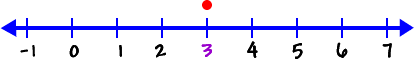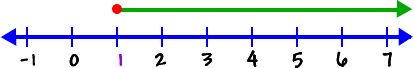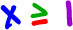For these problems, instead of just having one answer, like x = 3We are going to get a range of answers, called an interval...  Like this:This means that x can be 1 ... or x can be bigger than 1 ... x can be 2 or 4.671 or 1.00001 or 50,000,000.

One way to write this interval is(read "x is greater than or equal to 1" )

So, what would this mean on a number line?Just read it!  "x is less than 3."We put an open dot at the 3, since x cannot be 3.

So, x < 3 means that x cannot be 3, but it can be a number less than 3... like 2.998 ... or 0 ... or -5931.